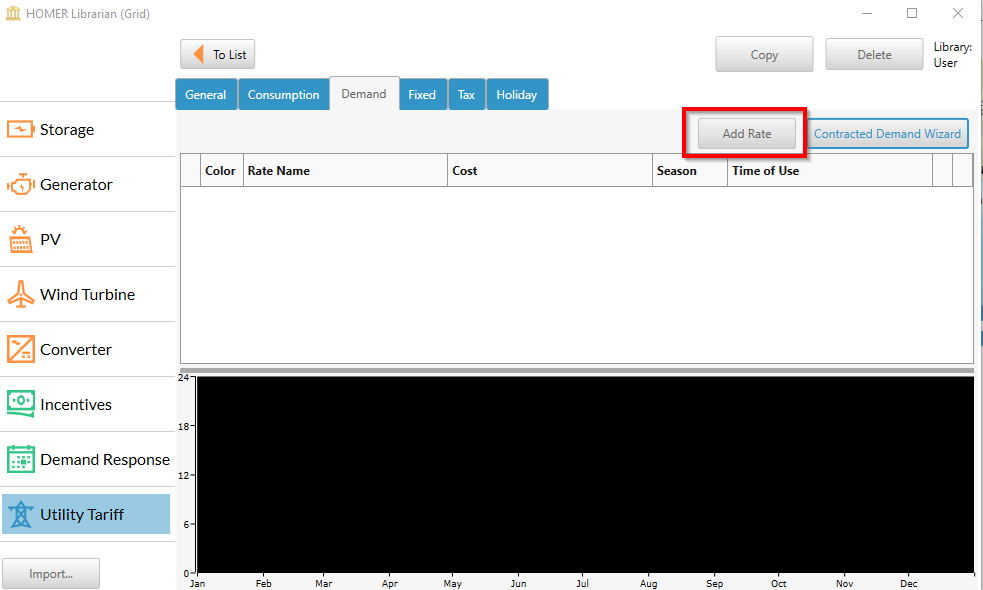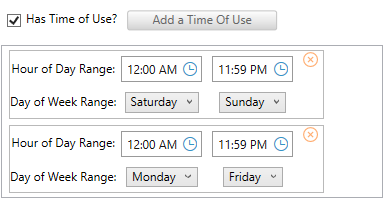HOMER Grid 1.6

 Demand RateYou can add multiple demand charges to your tariff. Click on the Add Rate button on the right corner under the demand tab.This will open up a pop-up below.Follow the below steps to add a demand rate.

Step 1: Enter the name of the demand rateStep 2: Choose the units of the demand rate.

The choices are: kW, kVA,and kVARStep 3: Specify the cost

1.Flat demand rate - If it is a constant energy rate (\$/kW) then enter the price in box for cost2.Tiered energy rate- If it is a tiered energy rate, then check the box that asks “Has Tiers”. This would display a table for you to enter the tiers and their costs. Enter the Limits for each Tier and the costs in \$/kWh. The table in the picture below, has the following tiers - from 0 to 50 kW, the rate is 5 \$ per kW. Between 50 -100 kW the rate is 10 \$ per kW. Any consumption above 100 kW would be charges 20 \$ per kW.

So if a customer consumed 300 kW in the billing period, then the cost = ( 50 kW * 5 \$/kW) + (100 kW *10 \$/kW) + (150 kWh * 20 \$/kW) = 4250 \$Step 4: Specify the Period

a.Select the interval over which the utility calculates the peak demand. The options are 15-, 30- or 60-minute peak demand intervals.

Note: If you are using a higher resolution load (for example, 15 minute interval data), but have kept the peak demand interval to 60 minutes, HOMER will average the load over 60 minutes to determine the peak. If you select a peak demand interval to be sub-hourly, use load data which has the same time-step length.b: If this rate to only apply during the summer season, then change the FROM and TO dates accordingly.c: The default demand rate is a monthly demand rate. This means that a new peak demand is calculated every month, and that is the amount for which the customer gets charged. If this demand rate considers a ratchet peak, then select the box named "Has Ratchet?"i: If you chose “Has Ratchet”, then you need to specify the ratchet period over which the peak demand is calculatedThe example above shows a summer ratchet, where the peak is calculated from the months of May 1st - Aug 31st. This peak is applied for the whole year. So every month, irrespective of what the monthly peak demand is, the customer is charged based on the ratchet peak.

d: If this rate has a Time of Use, then you could check the box that says “Has Time of Use”, and this would allow you to add multiple times periods to this rate.Select a Range of TOU in Number of Days in a week, and the time during each of the selected days.

Step 5: Specify if this rate applies during HolidaysIf this is checked, it would mean that this rate would be applied to any grid purchases during the holidays. To add all the public holidays in your region, go to the Add Holidays Section.

The Homer Support site has a searchable knowledgebase and additional support options. HOMER Online contains the latest information on model updates, as well as sample files, resource data, and contact information. ©2017 HOMER Energy, LLC